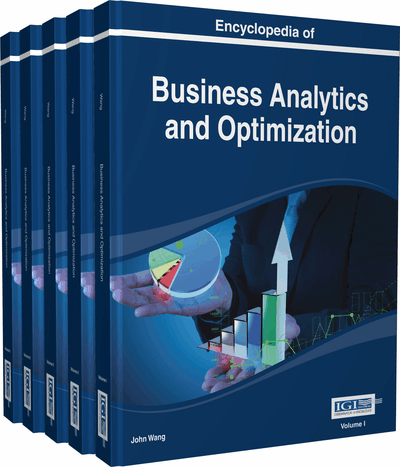# Support Vector Machine Models for Classification

Minghe Sun (College of Business, University of Texas at San Antonio, USA)
DOI: 10.4018/978-1-4666-5202-6.ch215

## Abstract

As machine learning techniques, support vector machines are quadratic programming models and are recent revolutionary development for classification analysis. Primal and dual formulations of support vector machine models for both two-class and multi-class classification are discussed. The dual formulations in high dimensional feature space using inner product kernels are emphasized. Nonlinear classification function or discriminant functions in high dimensional feature spaces can be constructed through the use of inner product kernels without actually mapping the data from the input space to the high dimensional feature spaces. Furthermore, the size of the dual formulation is independent of the dimension of the input space and independent of the kernels used. Two illustrative examples, one for two-class and the other for multi-class classification, are used to demonstrate the formulations of these SVM models.
Chapter Preview
Top

## Introduction

A support vector machine (SVM) is a quadratic programming (QP) model with training or learning algorithms. Developed by Vapnik (1995, 1998) and his coworkers, SVMs are used for classification, regression and function approximation. Because SVMs are QP models, this chapter discusses SVMs for classification from the mathematical programming (MP) perspective. SVMs are machine learning techniques because a SVM is usually trained, usually through numerical methods, to determine the values of the parameters in the classification function or discriminant functions.

As any other classification techniques, a SVM is used to construct a classification function or discriminant functions based on known values of the attributes or variables and known class memberships of the observations in a sample. The constructed classification function or discriminant functions are then used to evaluate the attribute values of any observation to obtain discriminant scores and to assign the observation into one of the classes. Many discriminant and classification techniques have been developed because no single technique always outperforms others under all situations (Johnson & Wichern, 1988). Statistical techniques, such as Fisher’s linear discriminant function (Fisher, 1936), Smith’s quadratic discriminant function (Smith, 1947) and logistic regression (Hand, 1981), have been standard tools for this purpose. More recently, other techniques, such as MP (Hand, 1981; Sun, 2010, 2011, 2014), neural networks (Stern, 1996) and classification trees (Breiman et al., 1984), have become alternative tools.

SVM (Vapnik, 1995, 1998) is a recent revolutionary development in classification analysis. Although they are modifications of the linear programming (LP) or mixed integer programming (MIP) models, they perform much better than their LP and MIP counterparts. Because they are QP models, they are also much more difficult to solve than their LP counterparts. Three concepts, classification margin maximization, dual formulation and kernels, are crucial in SVMs (Bredensteiner & Bennett, 1999). By minimizing the sum of classification errors and maximizing the classification margin at the same time in a QP model, SVMs construct discriminant functions with good generalization capabilities. Usually the dual formulation of the QP models is solved because the dual is usually easier to solve than the primal. By using inner product kernels in the dual formulations, SVMs can be built and nonlinear discriminant functions can be constructed in high dimensional feature spaces without carrying out the mappings from the input space to the high dimensional feature spaces. The size of the dual formulation is independent of the dimension of the input space and independent of the inner product kernels used.

However, most of the SVM research is for two-class classification although efforts have been made to extend the techniques to multi-class problems. Some of the approaches proposed in the literature for multi-class classification include the one-against-one approach (e.g., Friedman, 1996; Kreβel, 1999; Mayoraz & Alpaydin, 1999; Angulo et al., 2003), the one-against-all approach (e.g., Corte & Vapnik, 1995; Vapnik, 1995, 1998) and the one model formulation (e.g., Vapnik, 1995, 1998; Corte & Vapnik, 1995; Bredensteiner & Bennett, 1999; Weston & Watkins, 1999; Guermeur et al., 2000; Crammer & Singer, 2001; Guermeur, 2002; Lee et al., 2004; Sun, 2013). Examples of popular training software packages for SVMs include LIBSVM (Chang & Lin, 2011) and LIBLINEAR (Fan et al., 2008).

## Key Terms in this Chapter

Mathematical Programming: Mathematical models and associated solution techniques used to find one or more optimal solutions within a set of restrictions based on one or more criteria. A typical mathematical programming model has one or more objective functions, a number of decision variables and a set of constraints.

Support Vector Machine: A machine learning technique using quadratic programming models and solution techniques to analyze data and to construct functions for the purposes of function approximation, regression, classification and pattern recognition.

Primal Formulation: The primal formulation of a mathematical programming problem is the original model to be solved.

Machine Learning: Machine learning is a branch of artificial intelligence that constructs systems, such as mathematical equations, from available data and generalizes the constructed system to new data.

Classification Analysis: Constructing classification or discriminant functions to separate objects in two or more classes using variables measuring the characteristics of the objects and assigning new objects to the classes by evaluating the values of the variables measuring the objects using the constructed classification or discriminant functions.

Dual Formulation: The dual formulation of a mathematical programming problem is the mirror formulation of the primal formulation. The optimal value of the objective function of one provides a bound for that of the other. For general convex optimization problems, the optimal values of the objective functions of the primal and dual formulations are the same. If one formulation is unbounded, the other is infeasible. The dual formulation of the dual formulation is the primal formulation.

## Complete Chapter List

Search this Book:
Reset